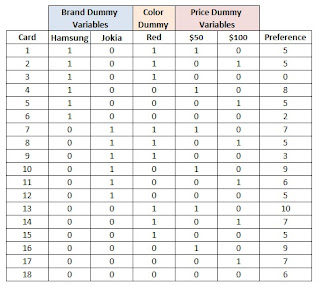## Pages

### How to perform Conjoint Analysis

First of all the categorical variables in data prepared for 18 mobiles in previous shown format is transformed to dummy variables :Normal data

Initial Format of Data >>>

Dummy Format of Data >>>Using the golden rule for creating dummy variables that if there are n variation in a categorical variables, we should make only n-1 dummy variables for it.

Hence the redundant variables highlighted in yellow in "Data with dummies" are removed and we are left with the data shown right >>>

With the use of Data Analysis Tools pack within Excel, we run a Regression analysis on the above data with Preference as dependent (Y) variables and Hamsung, Jokia, Red, \$50 and \$100 as independent variables (X variables).

### Result of Regression in Excel

The beta coefficients of the regression equation are called "Part Worth Utility" (PWU) of the variants of variables. Large part-worth utilities are assigned to the most preferred levels, and small part-worth utilities are assigned to the least preferred levels.

The PWU of \$50 is more than that of \$100, means that the buyer prefers \$50 mobile more that \$100 mobile. But I would suggest you to be little patient with interpretation, wait for utility calculations.

If A, B, C are the variants of a variable, and if the dummies A and B are used in model, the coefficient of A and B are both relative to C,  C's part worth utility is consider as "0" and hence the coefficients are part worth utility of A and B.

### Now we calculate the utility of each variant using part worth utilities.

As stated above : the coefficient of A and B are both relative to C, we can consider solving following sets of equations:

Set 1:
EQ 1 :    Hamsung - Pineapple = -3.17
EQ 2 :    Jokia - Pineapple = -1.50
EQ 3 :    Hamsung + Pineapple + Jokia  = 0

Set 2:
EQ 1 :    Red - Blue = -1.11
EQ 2 :    Red + Blue = 0

Set 3:
EQ 1 :    \$50  - \$150 = 4.50
EQ 2 :    \$100 -\$150 = 2.33
EQ 3 :   \$50 + \$100 + \$150 = 0

Solving above sets of equation gives the utility of individual variant of each variable.

Now you can start interpreting the choice and preferences of buyer.

1. Brand preference of Pineapple > Jokia > Hamsung
2. Color preference Blue > Red
3. Price preference \$50 > \$100>\$150 ...

### But one question is still unanswered ? What  ????

Which is more important for buyer : Brand, Color or Price ? How much weightage he gives to these attributes ?

So Now let's calculate Relative Importance Weights :

First we calculate the range of utility using following formula :

Range =  Maximum (Utility of variants) -   Minimum (Utility of variants)

Now relative importance or weighatge :

Importance of Brand  = range of Brand /  (Range of Brand + Range of Color + Range of Price )
Importance of Color  = range of Color /  (Range of Brand + Range of Color + Range of Price )
Importance of Price  = range of Price /  (Range of Brand + Range of Color + Range of Price )

With this I conclude the Linear Conjoint Analysis theoretical part. In subsequent article, I would explain the short and simple method to perform a conjoint analysis in SAS.

Enjoy reading our other articles and stay tuned with ...

Kindly do provide your feedback in the 'Comments' Section and share as much as possible.

#### 1 comment:

1.Thank you very much...you have stated it clearly in a simple manner...it helped me a lot for my thesis work...thanks

Do provide us your feedback, it would help us serve your better.